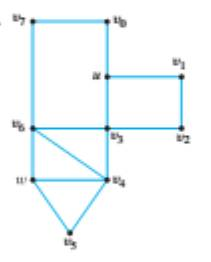Chapter 10.1, Problem 21ES### Discrete Mathematics With Applicat...

5th Edition
EPP + 1 other
ISBN: 9781337694193

#### Solutions

Chapter
Section### Discrete Mathematics With Applicat...

5th Edition
EPP + 1 other
ISBN: 9781337694193
Textbook Problem
1 views

# For each of the graph in 19-21, determine whether there is an Euler trial from u to w. If there is, find such a trail.To determine

For the graph, determine whether there is an Euler path rom u to w. If there is, find such a path.

Explanation

Given information:

Calculation:

The graph is connected degrees of u and w are odd and the degrees of other vertices are even that is why the graph has an Euler path.

The graph has an Euler path, which is given by uv0v7

### Still sussing out bartleby?

Check out a sample textbook solution.

See a sample solution

#### The Solution to Your Study Problems

Bartleby provides explanations to thousands of textbook problems written by our experts, many with advanced degrees!

Get Started